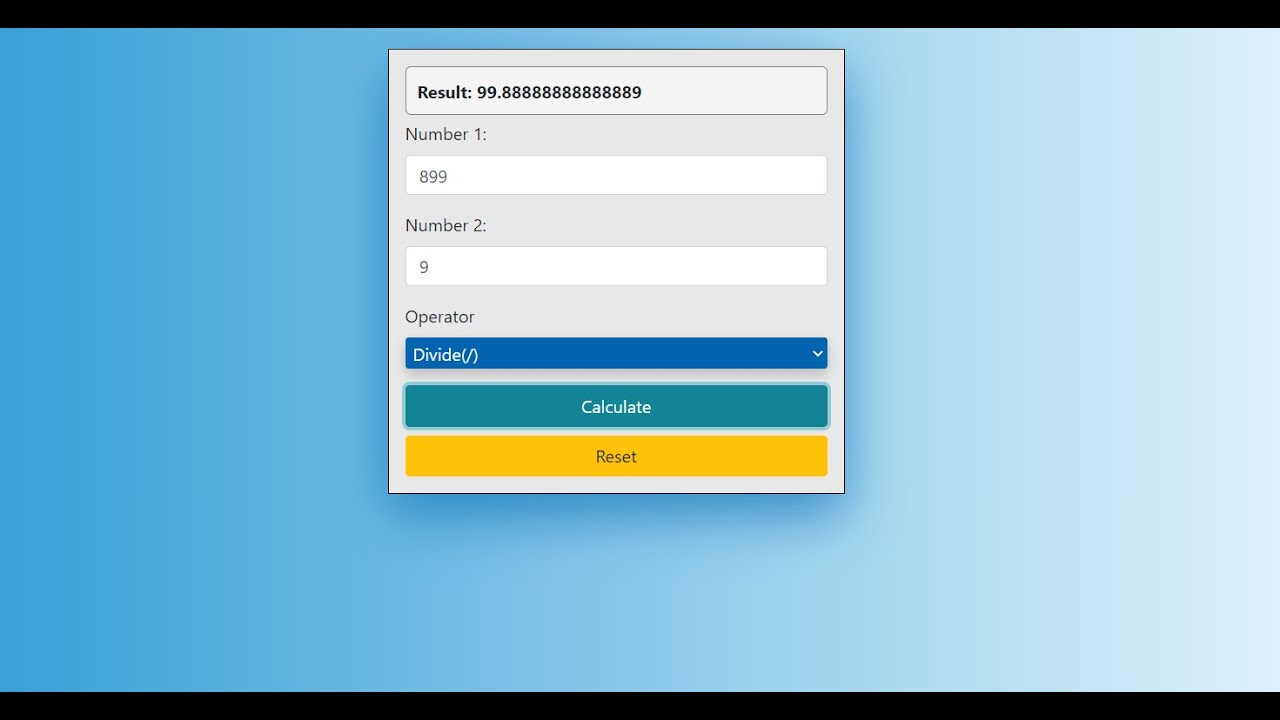# Simple JavaScript Calculator With Source CodeThis Simple JavaScript calculator project is a simple project in HTML5, CSS, and JavaScript. Here, the calculator functions as that of a real-life simple calculator. This project is for solving the mathematical calculations of numbers. You can see the basic features of the calculator in this project.

This Simple JavaScript calculator project is a simple project in HTML5, CSS, and JavaScript. Here, the calculator functions as that of a real-life simple calculator. This project is for solving the mathematical calculations of numbers. You can see the basic features of the calculator in this project.

## What are the differences between the various JavaScript frameworks? E.g. Vue.js, Angular.js, React.js

What are the differences? Do they each have specific use contexts?

## Ember.js vs Vue.js - Which is JavaScript Framework Works Better for You

In this article we will discuss full details and comparison of both Ember.js and Vue.js

## Grokking Call(), Apply() and Bind() Methods in JavaScript

In this article, we will have a look at the call(), apply() and bind() methods of JavaScript. Basically these 3 methods are used to control the invocation of the function.

## Top Vue.js Developers in USA

Vue.js is an extensively popular JavaScript framework with which you can create powerful as well as interactive interfaces. Vue.js is the best framework when it comes to building a single web and mobile apps.